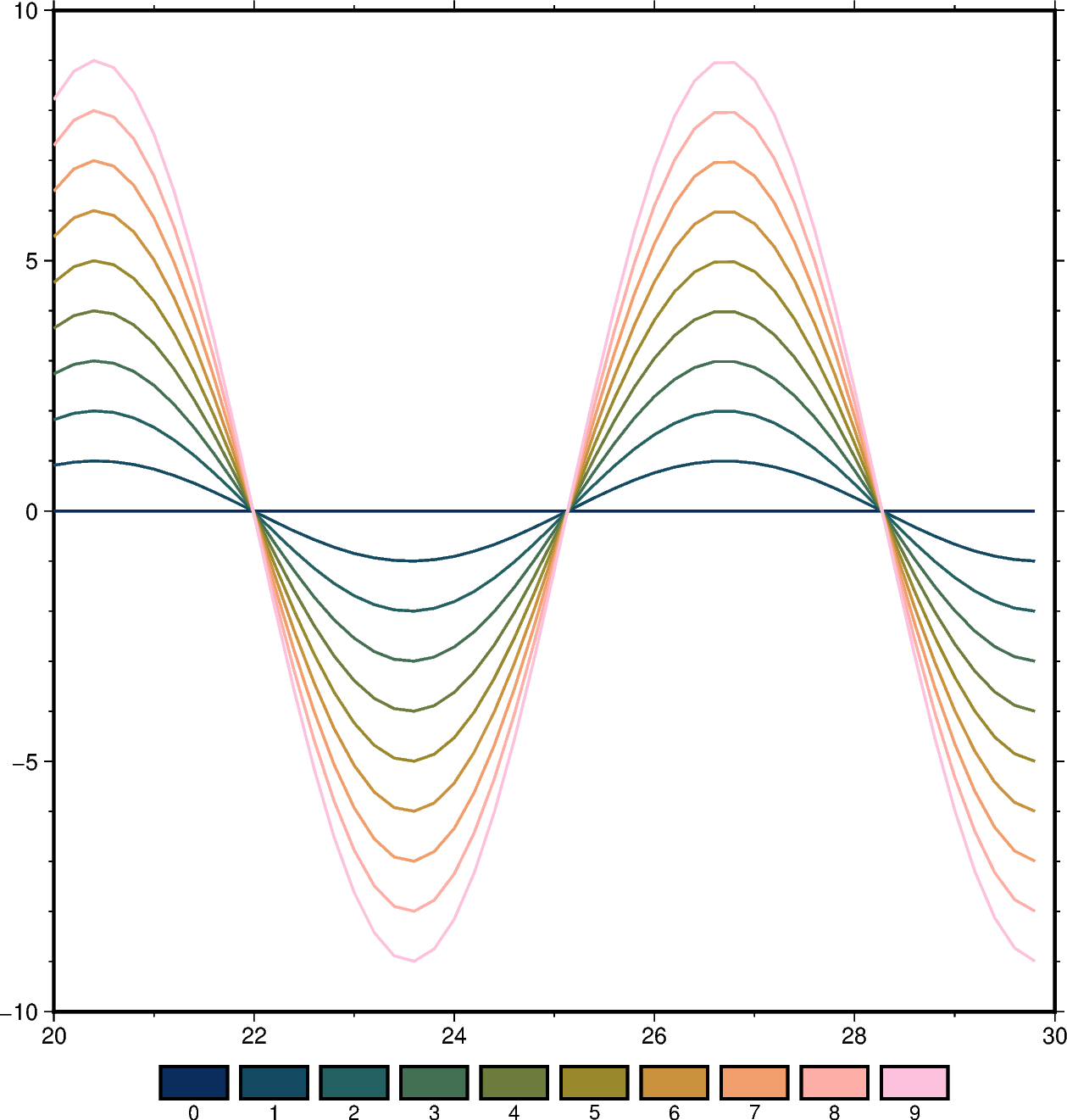# Line colors with a custom CPT

The color of the lines made by `pygmt.Figure.plot` can be set according to a custom CPT and assigned with the `pen` parameter.

The custom CPT can be used by setting the plot command’s `cmap` parameter to `True`. The `zvalue` parameter sets the z-value (color) to be used from the custom CPT, and the line color is set as the z-value by using +z when setting the `pen` color.```import numpy as np
import pygmt

# Create a list of values between 20 and 30 with 0.2 intervals
x = np.arange(start=20, stop=30, step=0.2)

fig = pygmt.Figure()
fig.basemap(frame=["WSne", "af"], region=[20, 30, -10, 10])

# Create a custom CPT with the batlow CPT and 10 discrete z-values (colors),
# use color_model="+c0-9" to write the color palette in categorical format and
# add labels (0) to (9) for the colorbar legend
pygmt.makecpt(cmap="batlow", series=[0, 9, 1], color_model="+c0-9")

# Plot 10 lines and set a different z-value for each line
for zvalue in range(0, 10):
y = zvalue * np.sin(x)
fig.plot(x=x, y=y, cmap=True, zvalue=zvalue, pen="thick,+z,-")

# Color bar to show the custom CPT and the associated z-values
fig.colorbar()
fig.show()
```

Total running time of the script: ( 0 minutes 1.625 seconds)

Gallery generated by Sphinx-Gallery